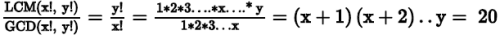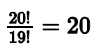For an integer n greater than 1, n! denotes t...

### Related TestFor an integer n greater than 1, n! denotes the product of all integers from 1 to n, inclusive. If x and y are two distinct positive integers such that y > x, what are the values of x and y?
(1) The ratio of the lowest common multiple and the highest common factor of y! and x! is 20:1.
(2) The lowest common multiple and the highest common factor of y! and x! have 3 and 2 prime factors respectively
• a)
Statement (1) ALONE is sufficient, but statement (2) alone is
• b)
Statement (2) ALONE is sufficient, but statement (1) alone is
• c)
BOTH statements (1) and (2) TOGETHER are sufficient to
• d)
EACH statement ALONE is sufficient to answer the question
• e)
Statements (1) and (2) TOGETHER are NOT sufficient to
problem are needed.ARJUN JAGMOHAN SINGHStep 1 & 2: Understand Question and Draw Inference
• n! = 1*2*3……*n
• x, y are distinct integers > o
• y > x
To Find: Values of x and y
Step 3 : Analyze Statement 1 independent
1. The ratio of the lowest common multiple and the highest common factor of y! and x! is 20:1.
• x! = 1*2*3…..*x
• y! = 1*2*3….x*(x+1)…….* y ( as y > x)
• So, we can write: y! = x! * a, where a is a positive integer
• As y! is a multiple of x!, LCM( x!, y!) = y!
• Similarly, as x! is a factor of y!, GCD(x!, y!) = x!
So,Hence, 20 needs to be expressed as a product of 1 or more consecutive positive
integers. Following cases are possible:
• As 20 = 22 * 5, following cases are possible:
• 20 = 4 * 5
• So, (x+1) * y = 20. Therefore, x + 1 = 4, i.e. x = 3 and y = 5
• The other possible case is when (x+1) = y = 20, i.e. y = 20 and x = 19. So,As we do not have unique values of x and y, the statement is insufficient to answer
Step 4 : Analyze Statement 2 independent
2. The lowest common multiple and the highest common factor of y! and x!
have 3 and 2 prime factors respectively.
• we know that LCM(x!, y!) = y! = 1*2*3*4*5…y (we deduced this in the analysis of statement-1)
• As y! has 3 prime factors only, they have to be the smallest 3 prime factors, i.e. {2, 3 , 5}. So, 5! ≤ y! < 7! as 7! will have 4 prime factors {2, 3, 5, 7}
• 5 ≤ y < 7. So, y = 5 or 6
• We also know that GCD(x!, y!) = x! = 1*2*3… x (we deduced this in the analysis of statement-1)
• As x! has 2 prime factors only, they have to be the smallest 2 prime factors , i.e. {2, 3}. So, 3!≤ x! < 5!, as 5! will have 3 prime factors {2, 3, 5}
• 3≤ x < 5 So, x = {3, 4}
As we do not have unique values of x and y, insufficient to answer
Step 5: Analyze Both Statements Together (if needed)
1. From statement-1, we inferred that y = {20, 5} and x = {19, 3}
2. From statement-2, we inferred that y = { 5, 6} and x = { 3,4 }
Combining both the statements, we have y = 5 and x = 3.View courses related to this question Explore GMAT courses
 Explore GMAT coursesView courses related to this question1 Crore+ students have signed up on EduRev. Have you?

• ### Directions: Each GMAT Data Sufficiency problem consists of a question and t... more(Scan QR code)